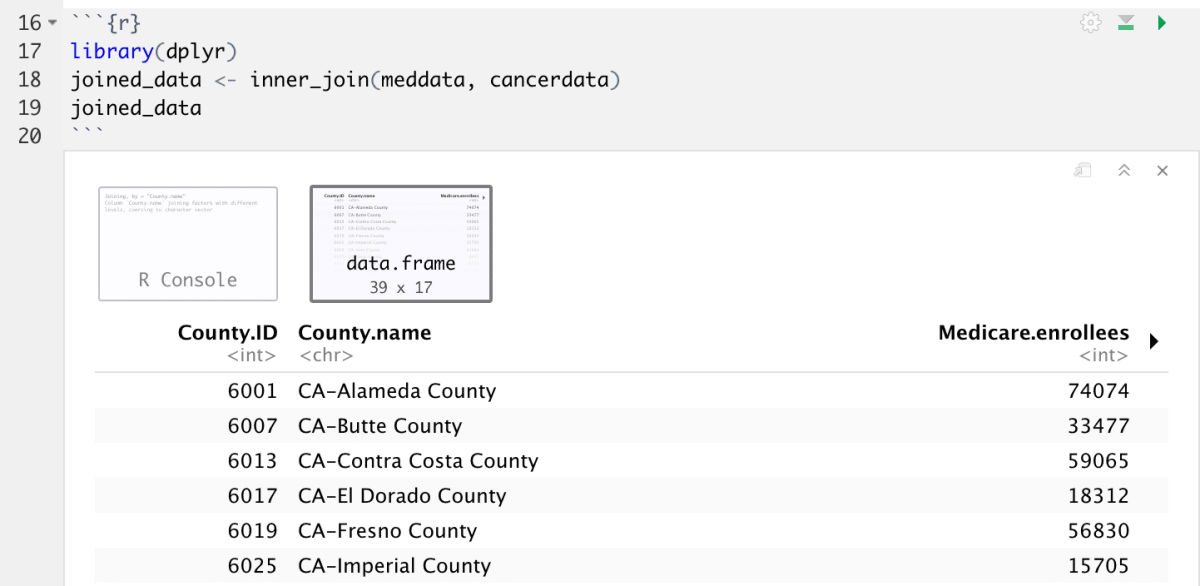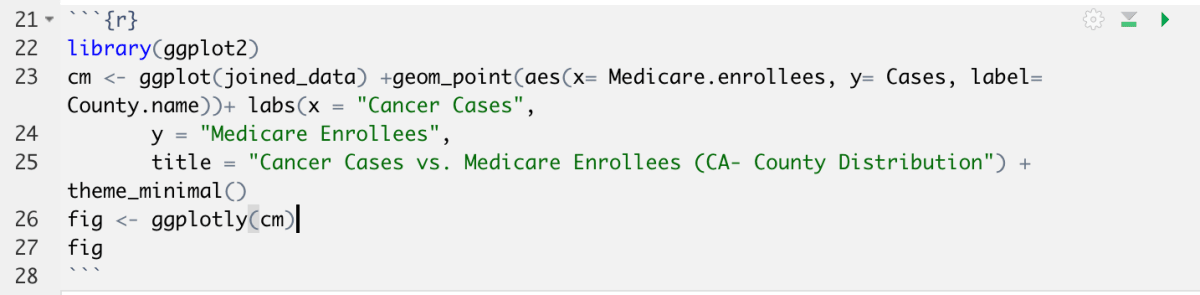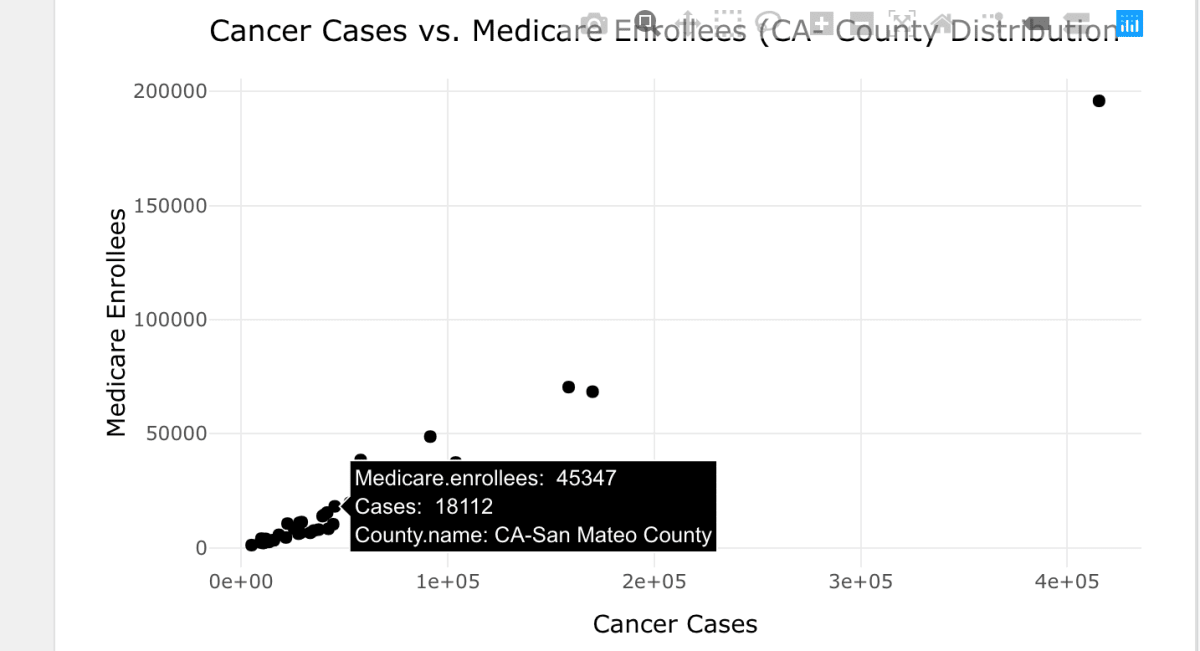# Data Wrangling

Data wrangling is the process of taking data in the raw form and converting it to something that is more understandable. Believe it or not, data scientists spend up to 60-80% of their time doing just this. Some operations in the process of data wrangling include spotting variables in the data, deriving new variables, reshaping the data, joining multiple datasets and creating group-wise summaries of the data.

## Example 1: Conducting ANOVA

In this example, we are going to be performing the ANOVA. I don’t want to dive too deep into stats so I shall leave you with this definition.

ANOVA: a statistical test that is designed to compare the means of more than two populations.

### Understanding the Data:

Note: we used this data in an earlier example. You may run through the next few lines as a refresher or skip ahead to the ‘Objective‘ section if you are familiar with the what the data describes.

We are going to be using the `Cushings` dataset from the `MASS` package. Please load in this library to your working environment.

Cushing’s syndrome is a hormonal disorder associated with an excessive secretion of cortisol from the adrenal gland.

Let’s take a look at the data.

```View(Cushings)
```The data contains 27 observations. For each observation, the urinary excretions rate of Tetrahydrocortisone(mg/24 hr) and Pregnantriol (mg/24 hr) are recorded. The letters a, b, c and u specify the underlying type of syndrome (i.e. adenoma, bilateral hyperplasia, carcinoma or unknown).

Objective: We are conducting ANOVA to find out whether there is a difference in the excretion rate of Tetrahydrocortisone between each type of type of syndrome.

X = Type of syndrome

 X Condition Number of observations 1 a adenoma 6 2 b bilateral hyperplasia 10 3 c carcinoma 5 4 u unknown 6
• X=1 : ‘a’ – adenoma
• X=2 : ‘b’ – bilateral hyperplasia
• X=3 : ‘c’ – carcinoma
• X=4 : ‘u’ – unknown

Y = Urinary excretion rate of Tetrahydrocortisone.

In more simplified terms, our objective is testing how much changes for different values of X.

To see the overall distribution of our data, we can use the summary function.

```summary(Cushings)
```We can see the range, mean, median and quartiles of our Tetrahydrocortisone levels to gain an understanding of the distribution.

Next let’s look at the means of each group using the group_by, summarise and mean functions.

```Cuchings_db <- Cushings %>%
select(Tetrahydrocortisone, Pregnanetriol, Type) %>%
group_by(Type) %>%
summarise(Tetrahydrocortisone = mean(Tetrahydrocortisone), Pregnanetriol = mean(Pregnanetriol))
Cuchings_db
```From this table, we can see that there is a striking difference between the Tetrahyrocortisone levels for each type of syndrome. Adenoma has the smallest mean of 2.96 while carcinoma has the largest mean, 19.72. We can compare these values to the overall mean from the summary function above, 10.46. It is evident that there is a striking variation in the excretory levels of Tetrahydrocortisone. Now let’s try to visualize and quantify this difference.

From our larger dataset, let’s subset the columns we are looking at and create a new data frame.

```type <-Cushings\$Type
tetra <-Cushings\$Tetrahydrocortisone
subset <- data.frame(type, tetra)
```

To plot the distribution of Tetrahydrocortisone, you may use the plot function.

```Cushings\$Tetrahydrocortisone
plot(type, tetra, main="Tetrahydrocortisone by Syndrome Type", xlab="Type", ylab="Tetrahydrocortisone")
```Plot is a useful function to plot two variables against one another. In this example, as there are multiple values for each combination ((Type a, Tetrahydrocortisone), (Type b, Tetrahydrocortisone)…), the function displays the information as a box plot.

From our boxplots, we can see that that is is quite a bit of variation amongst means in each type.

• This can be observed from the middle lines in the box plots and their varying heights

The range of values of each type also seems to have quite a bit of variation.

• This can be visualized in the difference in width of the box plots of ‘a’ and ‘u’

Both of these sources of variation contribute to the total variation of the observations around the mean.

To quantify the variation among the means of each syndrome, let’s conduct the ANOVA test using the `aov` function.

```aov.tt <- aov(tetra ~ type, data = subset)
aov.tt
```The first argument is a formula specifying the variables we would like to analyze variance within. The second is the dataset we are deriving values from.

ANOVA results are most commonly interpreted from ANOVA tables. The summary function creates the ANOVA table.

### Interpretation:

The first column shows the degrees of freedom (Df), which are k − 1 = 3 and n − k = 23, respectively. The values of the second column, labeled Sum Sq, are the variations between groups, SSB = 893.5 and variation within groups, SSW = 2123.6. The observed value of F -statistic is f = 3.2 given under the column labeled F value. The resulting p-value is then 0.04.  Essentially, the larger the F statistic, the larger the variation between groups. The p value is probability of getting an F value this large. A smaller p value tells us that the chances getting this F value are pretty slim. As our p value is less than 0.05, we can say that there is a significant variation for the Tetrahydrocortisone values for each type of condition.

This is a simplified version of the implications of the ANOVA test. If it sparks your interest, feel free to watch a few videos about the statistics involved to gain a more in depth understanding.

## Example 2: Joining Data and Visualization `

For those of you that completed the Python module, this data is going to look very familiar. For those of you that haven’t, heres a short description of the data:

• `med_county.csv`: My first dataset contains information about the medicare enrollees per county in the US
• `cancermodified.csv`My second dataset contains information about the incidence of cancer per county in California. This dataset is a modified version of the dataset I found online. It was modified to contain an extra column, that is now common to the med_county.csv data.

```install.packages("googledrive")
drive_deauth()### Converting Raw Data to a Useable Format

When I retrieved these datasets from a public database, they were both excel files. I had to do quite a bit of manipulation to get them to a useable format. You may come across a similar problem when retrieving data. I’ve compiled a quick list of tips to help you out in that situation.

• Open the data using a text editor. I used the built in application in my mac. Excel is a little deceptive in its display, so data that looks very clean, may have some flaws that would send you into a never ending circle of errors.• Understand your file format. E.g. a csv file contains a header and each value is separated by commas. My medicare dataset contained a lot of metadata at the top that deflected from this format. I later removed to make it easier to work with. Alternatively, I could have skipped the first few rows. Understanding how you data looks like and understanding the goal will make things a lot easier for you.
• Formatting your data. When I downloaded the data, numbers in the thousands range looked like this `2,000` . My desired format of a csv is going to misinterpret that number because it thinks that commas delimit my data. I had to format the data in excel to change these numbers to look like this `2000`### Joins and other functions

Joining is the process of combining two sets of data. There are a few methods of performing join. When describing the datasets, I mentioned that in the Python module, I modified the Cancer Incidence dataset to contain an extra column. This column is common to the Medicare Dataset. The reason behind this was to perform an inner join on the data.

Here is a quick explanation of the types of joins:To understand the concept of joins with respect to a table, let’s work through an example.

Dataset 1:

 S.No Code 1 ABA 2 AAA

Dataset 2:

 S.No UID 1 123 4 342

Inner join: This join is done based on a common parameter between the two sets of data. Only the data that is connected by the common element will be present in the new joined dataset

 S.No Code UID 1 ABA 123

The joined table would contain only the details of S.No 1, as it is the common element among the two.

Outer join: This type of join combines all the data that is present. If an element in the table is not present, the data is filled in with an NA. In this case, the elements 2 and 4 have some incomplete information. The data that is known is completed, the rest will be autofilled with an NA. In an outer join there is no loss of information.

 S.No Code UID 1 ABA 123 2 AAA NA 4 NA 342

Left Join: In left joins, the 1st table is printed entirely. Only the element that is common from the second dataset is printed in the table. As S.No 4 isn’t common to the first table, it is omitted entirely.

 S.No Code UID 1 ABA 123 2 AAA NA

Right Join: Right joins are very similar to left joins. The second dataset is printed entirely, and common elements from the first dataset are added. S.No 2 is omitted as it isn’t common to the second dataset.

 S.No UID Code 1 123 ABA 4 342 NA

### Joining Our Data:

Let’s apply this concept to our two sets of data.

To begin with, I set my working directory and load in my data.

```cancerdata <- read.csv('cancermodified.csv', header=TRUE, sep=",", strip.white=TRUE)
cancerdata
meddata
```The only difference from our normal process is the use of the `strip.white=TRUE` argument for our cancermodified.csv file. When viewing this file, I noticed the presence of white space between each column. This wasn’t the case with the med_county.csv file. When performing an inner join, I need my system to find an exact match between my two datasets. If not, it isn’t going to perform the join.

Essentially `[whitespace]CA-Butte County` isn’t going to match to `CA-Butte County`. The argument supplied helped me create that exact match.

Next, I performed the join using a function found in the `dplyr` library.

```library(dplyr)
joined_data <- inner_join(meddata, cancerdata)
joined_data
```Finally, I plotted a graph between two of the columns in my new joined dataset using the `ggplot2` and `plotly` libraries.

```library(ggplot2)
library(plotly)
cm <- ggplot(joined_data) +geom_point(aes(x= Medicare.enrollees, y= Cases, label= County.name))+ labs(x = "Cancer Cases",
y = "Medicare Enrollees",
title = "Cancer Cases vs. Medicare Enrollees (CA- County Distribution") + theme_minimal()
fig <- ggplotly(cm)
fig
```That marks the end of this module! I hope this module taught you some new concepts and skills in R and RStudio, that you can now apply to your own problems and data.